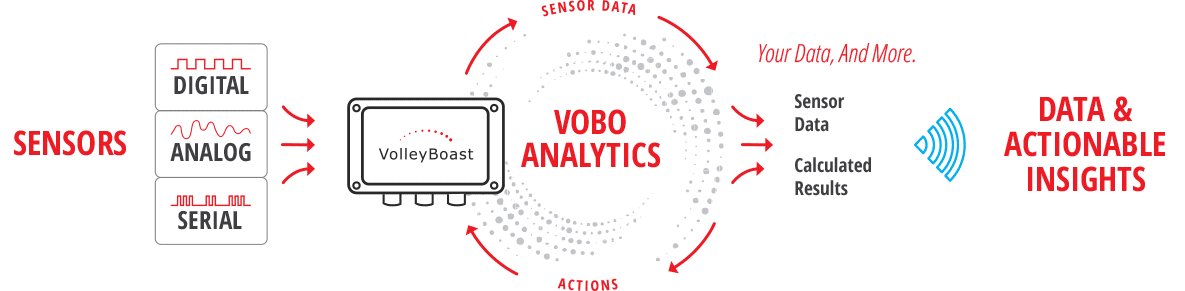## Unlock the intelligence inside your VoBo.

Add an analytics plug-in to your VoBo.  Run functions on the measurement data, bringing computational insights to the very edge.  The plug-in analytics calculates results based on a customer defined algorithm or select a function from the library of common analytics.  Algorithms can be applied to a single, multiple, or between multiple data channels, including Modbus RTU data.  Report analytical results in addition to, or in place of, the measured data.  The analytics function can also prompt the VoBo to initiate an action based on the results.  Actions could be transmitting data, changing a configuration parameter, or writing a value into a Modbus register.### Some of the many uses for the analytics plug-in are:

• Transmit data or send an indication that a condition is met, when a measured or calculated value exceeds or falls below a set or calculated value.
• Calculate average, mode, or standard deviation of measurement data channels.
• Calculate the rate of increase or decrease of a data channel.
• Perform calculations between multiple data channels.
• Collect measurement data at a higher frequency, calculate results, and report those results at a lower frequency.
• Collect pulse counts continuously for a short duration, convert to a measured quantity and report the calculated total.
• Change a VoBo configuration parameter when a certain calculation result is met. For example, when a pressure sensor reads above a set or calculated level, the frequency of the measurement is increased for as long as the pressure is above that level.

### Select from the library of common analytical functions, or work with us to develop an algorithm tailored to your application.  The library of functions contains computations for:

• Minimum Value
• Maximum Value
• Average Value
• Standard Deviation